MCAT Physical : Vapor Pressure

Example Questions

← Previous 1

Example Question #1 : Vapor Pressure

Boiling point is the temperature a liquid needs to achieve in order to begin its transformation into a gaseous state. Campers and hikers who prepare food during their trips have to account for differences in atmospheric pressure as they ascend in elevation. During the ascent, the decrease in atmospheric pressure changes the temperature at which water boils.

Further complicating the matter is the observation that addition of a solute to a pure liquid also changes the boiling point. Raoult’s Law can be used to understand the changes in boiling point if a non-volatile solute is present, as expressed here.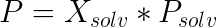In this law,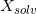is the mole fraction of the solvent,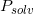is the vapor pressure of the pure solvent, andis the vapor pressure of the solution. When this vapor pressure is equal to the local atmospheric pressure, the solution boils.

If a non-volatile solute is added to a solvent, the freezing point of the solution tends to __________ relative to the pure solvent's freezing point.

increase

either decrease or increase

decrease

remain unchanged

be elevated only at low pressures

decrease

Explanation:

Freezing points are decreased, or depressed, with the addition of non-volatile solutes in a similar manner to boiling point elevation. The addition of a solute makes phase changes more difficult, and thus solutions with non-volatile solutes require more heat to boil, or a colder environment to freeze. The solute ions and particles in solution disrupt the forces between solvent molecules, preventing the formation of a solid frozen lattice.

Example Question #2 : Vapor Pressure

The addition of a nonvolatile solute to a solvent will raise its boiling point. This is because __________.

the solute increases the vapor pressure

the solute increases the heat capacity of the solvent

the solute reacts with the solvent and creates a product more resistant to temperature change

the solute causes more heat to be needed in order for the solution to have the same vapor pressure as the atmospheric pressure

the solute causes more heat to be needed in order for the solution to have the same vapor pressure as the atmospheric pressure

Explanation:

Remember that a nonvolatile solute will lower the vapor pressure of a solvent in proportion to the mole fraction of the solvent (Raoult's Law). Since vapor pressure must equal the atmospheric pressure in order to boil, a greater amount of heat is required to increase the lowered vapor pressure of the solution.

Example Question #3 : Vapor Pressure

A nonvolatile solute is added to a solution so that it makes up 5% of the molecules in the solution. Which of the following is true?

The vapor pressure is unaffected by the addition of the solute

The solution's vapor pressure is less than 95% of the pure solvent's vapor pressure

The solution's vapor pressure is somewhere between 95% and 100% of the pure solvent's vapor pressure

The vapor pressure of the solution is greater than the pure solvent's vapor pressure

The solution has 95% of the vapor pressure of the pure solvent

The solution has 95% of the vapor pressure of the pure solvent

Explanation:

When a nonvolatile solute is added to a solvent, it will not contribute to the molecules which exert pressure on the container. It will, however, take up some of the surface area interacting with the air in the container. This reduces the number of solvent molecules that are able to break from their bonds and become gas molecules in the container. The reduction of vapor pressure is dependent on the percentage of solute molecules in the solution. Since 5% of the molecules in this solution come from the solute, the vapor pressure will be 95% of the pure solvent's vapor pressure.

Example Question #4 : Vapor Pressure

Boiling point is the temperature a liquid needs to achieve in order to begin its transformation into a gaseous state. Campers and hikers who prepare food during their trips have to account for differences in atmospheric pressure as they ascend in elevation. During the ascent, the decrease in atmospheric pressure changes the temperature at which water boils.

Further complicating the matter is the observation that addition of a solute to a pure liquid also changes the boiling point. Raoult’s Law can be used to understand the changes in boiling point if a non-volatile solute is present, as expressed here.In this law,is the mole fraction of the solvent,is the vapor pressure of the pure solvent, andis the vapor pressure of the solution. When this vapor pressure is equal to the local atmospheric pressure, the solution boils.

A scientist is studying an unknown solution with a non-volatile solute, and determines that the solute has a mole fraction of 0.36. The original solvent has a vapor pressure of 2atm. What is the vapor pressure of the solution being studied?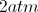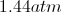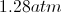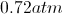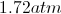Explanation:

The question gives us the mole fraction of solute, not solvent, thus, we need to realize that the mole fraction of solvent is the difference between one and the given value.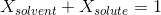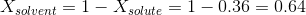Now we can use Raoult's Law to find the solution vapor pressure.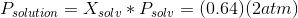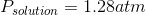Example Question #5 : Vapor Pressure

Boiling point is the temperature a liquid needs to achieve in order to begin its transformation into a gaseous state. Campers and hikers who prepare food during their trips have to account for differences in atmospheric pressure as they ascend in elevation. During the ascent, the decrease in atmospheric pressure changes the temperature at which water boils.

Further complicating the matter is the observation that addition of a solute to a pure liquid also changes the boiling point. Raoult’s Law can be used to understand the changes in boiling point if a non-volatile solute is present, as expressed here.In this law,is the mole fraction of the solvent,is the vapor pressure of the pure solvent, andis the vapor pressure of the solution. When this vapor pressure is equal to the local atmospheric pressure, the solution boils.

A scientist is testing Raoult's Law, but accidently adds a volatile solute instead of a non-volatile solute. Which of the following is true?

A volatile solute will not dissolve into solution

Vapor pressure may either increase or decrease compared to the pure solvent

Vapor pressure will remain unchanged compared to the pure solvent

Vapor pressure must decrease compared to the pure solvent

Vapor pressure must increase compared to the pure solvent

Vapor pressure may either increase or decrease compared to the pure solvent

Explanation:

A volatile solute has its own vapor pressure. As a result, it may lead to a higher total vapor pressure than the solvent in isolation. Since we are not told the vapor pressure of the volatile solute, it can either raise or lower the total vapor pressure. This will depend on the comparison of the vapor pressure of the pure solute and that of the pure solvent. If the solute has higher vapor pressure, then adding it to the solvent will raise the vapor pressure. If the solvent has higher vapor pressure, then adding solute will lower the vapor pressure.

Example Question #6 : Vapor Pressure

Boiling point is the temperature a liquid needs to achieve in order to begin its transformation into a gaseous state. Campers and hikers who prepare food during their trips have to account for differences in atmospheric pressure as they ascend in elevation. During the ascent, the decrease in atmospheric pressure changes the temperature at which water boils.

Further complicating the matter is the observation that addition of a solute to a pure liquid also changes the boiling point. Raoult’s Law can be used to understand the changes in boiling point if a non-volatile solute is present, as expressed here.In this law,is the mole fraction of the solvent,is the vapor pressure of the pure solvent, andis the vapor pressure of the solution. When this vapor pressure is equal to the local atmospheric pressure, the solution boils.

How would you best modify Raoult's Law to find the total vapor pressure of a solution with a volatile solute?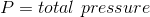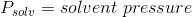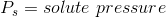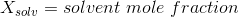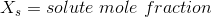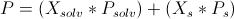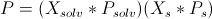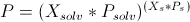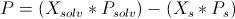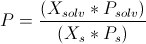Explanation:

You would add the contribution of the solute to the total vapor pressure of the solvent. Since the solute is contributing to the total vapor pressure of the solution, it must simply be added to the solvent vapor pressure.

Example Question #31 : Colligative Properties

Which statement is false with regard to vapor pressure?

The boiling point occurs when the vapor pressure of a liquid equals the local atmospheric pressure

Vapor pressure decreases when a non-volatile solute is added to a pure solvent

Substances with stronger intermolecular forces tend to have lower vapor pressures

Diethyl ether has a lower vapor pressure than water due to its greater molecular weight

Vapor pressure occurs in a state of dynamic equilibrium in which the rate of liquid converting to gas equals the rate of gas converting to liquid

Diethyl ether has a lower vapor pressure than water due to its greater molecular weight

Explanation:

Vapor pressure represents a state of dynamic equilibrium in which the rate of liquid escaping to gas is equal to the rate of gas condensing to liquid. Liquids with stronger intermolecular forces have a smaller amount of liquid escaping to gas, and thus have a lower vapor pressure.

Diethyl ether does not create hydrogen bonds, whereas water does. Even though diethyl ether has a greater molecular weight, it will have weaker intermolecular forces. The hydrogen bonding interactions in water will cause molecules to "stick," preventing them from converting to the gas phase and lowering the overall vapor pressure of water. Diethyl ether has a higher vapor pressure than water due to the intermolecular forces of the two compounds.

Example Question #32 : Colligative Properties

When a created solution is either exothermic or endothermic, the vapor pressure in the container will deviate from Raoult's law. As a result, the solution is considered non-ideal.

When a solute is added to a solvent, the vapor pressure of the solution is greater than the vapor pressure of either pure solute or pure solvent. Based on this, which statement is true?

The solution is exothermic

The intermolecular bonds in solution are stronger than the intermolecular bonds between the pure substances

The solution is considered ideal

There is a positive enthalpy of solution

There is a positive enthalpy of solution

Explanation:

Raoult's law can be written as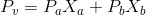.

When the formation of a solution has a positive enthalpy, it is considered to be endothermic. An endothermic reaction will result in the solution's vapor pressure being higher than predicted by Raoult's law. This is because an endothermic reaction results in weaker intermolecular bonds, which increases the vapor pressure.

Example Question #33 : Colligative Properties

A volatile solute with a vapor pressure of 80mmHg is added to a solvent with a vapor pressure of 120mmHg. Consider the resulting solution to be ideal in nature. The solution's vapor pressure is determined to be 93mmHg. What is the percentage of solute molecules found in the solution?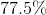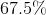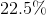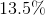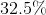Explanation:

A volatile solute will contribute to the vapor pressure found in the container. As a result, we have to use Raoult's law, which takes this solute's vapor pressure into consideration.

Raoult's law is written as, where P is the partial pressure for each component and X is the mole fraction of each component.

We must include the vapor pressures of both the solute and the solvent in order to find the percentage composition of the solute in the solution. Since there are only two compounds contributing to vapor pressure in the solution, we can designate the mole fraction of the solute as X and the mole fraction of the solvent as (1-X). Doing this, the equation can be filled in, as below.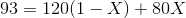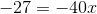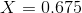or 67.5% solute in the container.

Example Question #34 : Colligative Properties

At room temperature, toluene has a vapor pressure of 300mmHg and ethanol has a vapor pressure of 45mmHg.

Let us assume that when equimolar amounts of these liquids are mixed together, heat is released. What can be said about the vapor pressure of the resulting solution?

The vapor pressure will be 345 mmHg because the vapor pressure values are additive

The vapor pressure will be 300 mmHg because toluene has the greater vapor pressure, which cancels out the vapor pressure of ethanol

The vapor pressure will be 172.5 mmHg because there are equal amounts of both liquids

The vapor pressure is less than 172.5 because the reaction is exothermic

The vapor pressure is less than 172.5 because the reaction is exothermic

Explanation:

Since both liquids are volatile, they will both contribute to the solution's vapor pressure. Their impact will be based on their individual vapor pressures, as well as the molar fraction for which each liquid is responsible. This gives us Raoult's law: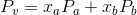Eachrepresents the liquid vapor pressure, and eachrepresents the corresponding molar fraction of that particular liquid. Since we have an equimolar mixture, each liquid accounts for 50% of the solution, or in this case 0.5.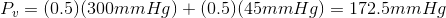This would result in a solution vapor pressure of 172.5 mmHg if conditions were ideal. However, since the reaction is exothermic, stronger bonds are formed in the solution, and the vapor pressure will be lower than expected.

← Previous 1